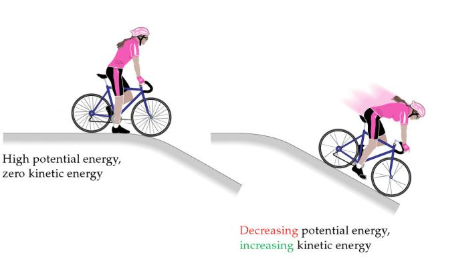# Problem: Potential energy and kinetic energy. The potential energy initially stored in the motionless bicycle at the top of the hill is converted to kinetic energy as the bicycle moves down the hill and loses potential energy.(b) When the cyclist and bicycle in the following figure come to a stop at the bottom of the hill, is the kinetic energy the same as it was at the top of the hill?

###### FREE Expert Solution

kinetic energy:

$\overline{)\mathbf{K}\mathbf{.}\mathbf{E}\mathbf{.}\mathbf{=}\frac{\mathbf{1}}{\mathbf{2}}{\mathbf{mv}}^{\mathbf{2}}}$

when the cyclist and bicycle in the following figure come to a stop at the bottom of the hill:

94% (403 ratings)###### Problem Details

Potential energy and kinetic energy. The potential energy initially stored in the motionless bicycle at the top of the hill is converted to kinetic energy as the bicycle moves down the hill and loses potential energy.(b) When the cyclist and bicycle in the following figure come to a stop at the bottom of the hill, is the kinetic energy the same as it was at the top of the hill?